AlgorithmsNext: AES algorithm Up: Algorithms and tie-point Previous: Algorithms and tie-point

Algorithms

In this section, the 5 used algorithms to retrieve ice concentration will be outlined.
Most algorithms are based on two simple equations:where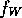,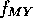and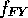are the amount of open water, multi-year ice and first-year ice, respectively.is not to be confused with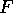from equation 1. The second formula is: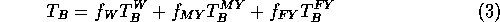where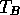is the brightness temperature and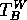,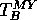and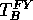are the brightness temperatures from open water, multi-year ice and first-year ice, respectively.
The algorithms will be addressed separately below.

Tim Flintholm Fink<tff@emi.dtu.dk>
Thu Oct 26 09:07:34 NFT 1995